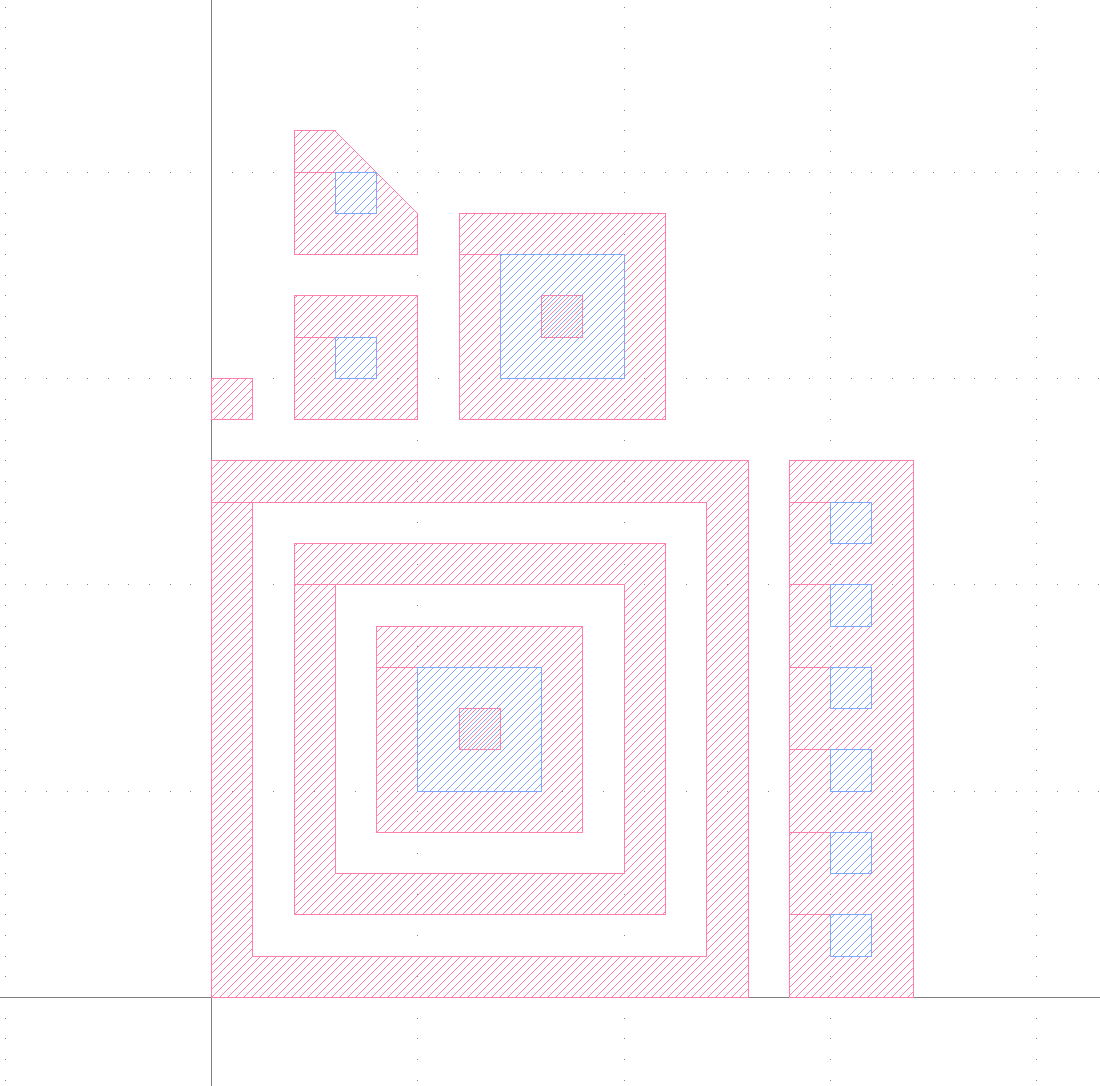# How to select "inner holes" by Boolean Operation in DRC modes?

edited September 17How can I reach the above result?

The "inner holes" are the holes that contain no any other hole inside.
My KLayout version is 25.0.5

``````        L1 = input(1,0)

L2 = L1.holes
L2.output(2,0)

L3 = L1.select{|polygon|
polygon.holes <= 1
}.holes
L3.output(3,0)

\$tmp = L1
while not (\$tmp.holes & L1).holes.is_empty?
\$tmp = \$tmp.holes & L1
end
L4 = \$tmp.holes
L4.output(4,0)

L5 = L1.holes.select_not_overlapping(L1 - L1.select{|polygon| polygon.is_box?})
L5.output(5,0)
``````

But L2, L3, L4 cannot reach the result I want.
I think I need exclude the L1 with no holes.
So, I come up with L5, but why it fail?
Is there any short Boolean operation could find all inner holes?

Thanks!

• edited September 19

@intervalrain That's an interesting challengeThere is no short boolean operation which does that. But I think this operation should do the job:

``````l1wh = L1.with_holes(1, nil)
inner_holes = (l1.holes - l1wh).without_holes(1, nil)
``````

Matthias

• Thank you, Matthias.

In Version 0.25.5,

I could reach the result with

`L1 = input(1,0)

l1wh = L1.select{|polygon|polygon.holes==1}
l1wh.output(2,0)
inner_holes = (L1.holes-l1wh).select{|polygon|polygon.holes==0}
inner_holes.output(3,0)`

• edited September 22

That's right. This is the equivalent code. But it's slower because the loop is going to be executed in the slow script interpreter.

BTW: why the old KLayout version?

I have noticed that the "(1, nil)" argument to "with(out)_holes" is redundant and not intuitive, so for 0.27.4 I will introduce a parameterless version of "with_holes" and "without_holes" which makes the expression even shorter:

``````l1wh = L1.with_holes
inner_holes = (L1.holes - l1wh).without_holes
``````

I do have a few more remarks:

• Don't use with_holes and without_holes in tiled mode as tile cutting may remove the "holeness". However, deep mode will work.
• For the layer names you should user lower-case names ("l1" instead of "L1") as Ruby (which is the basis of DRC scripts) regards all upper-case variables as constants and complains when you redefine them.

Regards,

Matthias